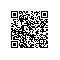# 插入排序

### 基本思想

，直到对象全部插入为止。

直接插入排序



### 直接插入排序void InsertSort（SqList &L）
{
int i，j；

  for(i=2;i<L.length;++i)

if(L.r[i].key<L.r[i-1].key)        //"<",需将r[i]插入有序子表
{
L.r=L.r[i];      //将待插入的记录暂时存在监视岗哨中

L.r[i]=L.r[i-1];     //r[i-1]后移

for(j=i-2;L.r.key<L.r[j].key;--j)//从后向前寻找插入位置
{

L.r[j+1]=L.r[j];//记录逐个后移，直到找到插入位置

}

L.r[j+1]=L.r;    //将r即原r[i]，插入到正确位置
}
}


#### 最好情况下：

• 每趟只需要比较1次，不移动

• 总比较次数为n-1#### 最坏情况下：第i趟比较i次，移动i+1次#### 算法分析

• 若出现各种可能排序的概率相同，则可取最好情况和最坏情况的平均情况
平均情况比较次数和移动次数为n*n/4

• 时间复杂度为O(n*n)

• 空间复杂度为O(1)

• 是一种稳定的排序方法

### 折半插入排序

(3)如此直至low<=high不成立，即将此位置之后所有元素后移一位，并将新元素插入a[high+1]

Void BInserSort(SqList &L)
{
for(i=2;i<L.Length;++i)//对顺序表L做折半插入排序
{
L.r=L.r[i];//将待插入的记录暂存到监视哨中
low=1;high=i-1;//置查找区间初值
while(low<=high)  //在r[low.high]中折半查找插入的位置
{
m=(low+high)/2   //折半
if(L.r.key<L.r[m].Key) high=m-1;//插入点在前一个子表
else low=m+1;      //插入点在后一子表
}
for(j=i-1;j>high+1;--j) L.r[j+1]=L.r[j];//记录后移
L.r[high+1]=L.r;   //将r即原r【i】，插入到正确位置
}

}


#### 算法分析

• 减少了比较次数，但没有减少移动次数

• 平均性能优于直接插入排序

• 时间复杂度为O(n*n)

• 空间复杂度为O(1)

• 是一种稳定的排序方法

• 只能用于顺序结构，不能用于链式结构

• 适合初始记录无序，n较大的情况

### 希尔排序

#### 基本思想：#### 优点：

• 小元素跳跃式前移

• 最后一趟增量为1时，序列已基本有序

• 平均性能优于直接插入排序

void ShellInsert(SqList &L,int dk)
{
for(i=dk+1;i<=L.length;++i)

if(L.r[i].Key<L.r[i-dk].key)
{
L.r=L.r[i];
for(j=i-dk;j>0&&L.r.key<L.r[j].key;j-=dk)
{
L.r[j+dk]=L.r[j];

}
L.r[j+dk]=L.r;
}

}

void ShellSort(SqList &L,int dt[],int t)
{
for(k=0;k<t;++k)
{

ShellInsert(L,dt[k]);
}
}使用钉钉扫一扫加入圈子
+ 订阅